﻿ DPSFHelper Methods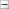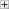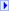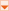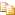Collapse AllExpand AllCode: All Code: Multiple Code: C# Code: Visual Basic Code: Visual C++Members: Show All Members: Filtered Members: Filtered Members: Filtered

[TCheckBoxFormControlObj]C#

[TCheckBoxFormControlObj]Visual Basic

[TCheckBoxFormControlObj]
Visual C++

[TCheckBoxFormControlObj]Include Protected Members

[TCheckBoxFormControlObj]
Include Inherited Members
 DPSF API Documentation DPSFHelper MethodsThe
DPSFHelper type exposes the following members.Methods

 Name DescriptionCloneBlendState Returns a clone of the given BlendState. Read-only properties, such as the GraphicsDevice property, are not copied though.CloneDepthStencilState Returns a clone of the given DepthStencilState. Read-only properties, such as the GraphicsDevice property, are not copied though.CloneRasterizerState Returns a clone of the given RasterizerState. Read-only properties, such as the GraphicsDevice property, are not copied though.CloneSamplerState Returns a clone of the given SamplerState. Read-only properties, such as the GraphicsDevice property, are not copied though.FadeInQuicklyAndFadeOutQuicklyBasedOnLifetime Returns how transparent a Particle should be, based on it's Normalized Elapsed Time, so that it fades in quickly and fades out quickly.FadeInQuicklyAndFadeOutSlowlyBasedOnLifetime Returns how transparent a Particle should be, based on it's Normalized Elapsed Time, so that it fades in quickly and fades out slowly.FadeInQuicklyBasedOnLifetimeFadeOutQuicklyBasedOnLifetimeInterpolationAmountForEqualLerpInAndLerpOut Returns the interpolation amount (between 0.0 and 1.0) that should be used in a Lerp function to have a property reach its full value when the NormalizedLifetime reaches 0.5, and go back to its original value by the time the NormalizedLifetime reaches 1.0. An example of where to use this would be if you wanted a particle to start off small, and reach its full size when the particle's lifetime is half over, and then to shrink back to being small by the time the particle dies. You would use the value returned by this function as the interpolation amount for the Lerp function. e.g. MathHelper.Lerp(SmallSize, LargeSize, ValueReturnedByThisFunction).LerpColor(Color, Color, Single) Returns the linearly interpolated Color between Color 1 and Color 2LerpColor(Color, Color, Single, Single, Single, Single) Returns the linearly interpolated Color between Color 1 and Color 2NormalizedPointOnSphere Returns a point on a sphere with a radius of one. To use a different radius simply multiply the returned value by the desired radius value, before translating it to the sphere's position in world coordinates. To create a circle simply use a constant value for one of the Angles while changing the other Angle.PointOnCircle Returns a point on a circle with a radius of one, on the X-Y axis plane. To use a different radius simply multiply the returned value by the desired radius value.PointOnCircleVector3 Returns a point on a circle with a radius of one, on the X-Y axis plane (Z value of zero). To use a different radius simply multiply the returned value by the desired radius value.PointOnSphere(Single, Single, Single) Returns a point on a sphere with the given Radius. To create a circle simply use a constant value for one of the Angles while changing the other Angle.PointOnSphere(Single, Single, Single, Vector3) Returns a point on a sphere with the given Radius, at the specified Sphere Position. To create a circle simply use a constant value for one of the Angles while changing the other Angle.RandomColor Returns a random opaque Color (i.e. no transparency)RandomColorWithRandomTransparency Returns a random Color with a random alpha value as well (i.e. random transparency)RandomNormalizedPointOnSphere Returns a random point on a sphere with a radius of one. To use a different radius simply multiply the returned value by the desired radius value, before translating it to the sphere's position in world coordinates.RandomNormalizedVector Returns a vector with a Random direction that has been Normalized.RandomNumberBetween Returns a random number between the specified valuesRandomPointOnCircle Returns a random point on a circle with a radius of one, on the X-Y axis plane. To use a different radius simply multiply the returned value by the desired radius value.RandomPointOnCircleVector3 Returns a random point on a circle with a radius of one, on the X-Y axis plane (Z value of zero). To use a different radius simply multiply the returned value by the desired radius value.RandomPointOnSphere(Single) Returns a random point on a sphere with the given RadiusRandomPointOnSphere(Single, Vector3) Returns a random point on a sphere with the given Radius, at the specified Sphere PositionRandomVectorBetweenTwoVectors Returns a Vector whose individual XYZ components are each randomly chosen to be somewhere between the two given Vectors' individual XYZ components. Unlike choosing a random Lerp value between two vectors, which would give a point somewhere on the LINE between the two points, this chooses a random Lerp value between each of the two vectors individual xyz components, returning a point somewhere in the cube-shaped AREA (i.e. Volume) between the two points.SourceToTargetDirection Returns a normalized vector representing the direction that points from the source point to the target point.SourceToTargetVector Returns a vector representing the line from the source point to the target point.ValueInRange Returns the given value, enforcing it to be within the given range.VectorsAreEqualWithinTolerance Returns true if the difference between the individual XYZ components of the given Vectors are all less than the specified ToleranceVectorsAreTheSamePolarity Returns if the polarity (i.e. positive or negative) of each X,Y,Z component of the two vectors are the same or not. Example: if Vector 1's X component is positive and Vector 2's X component is negative, this will return false. Example: if Vector 1's X,Y,Z components are all positive, and Vector 2's X,Y,Z components are all positive, this will return true.See Also

DPSFHelper Class

DPSF NamespaceSend comments on this topic to